Chapter 3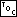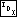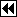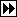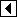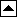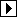#### Section 3.1

1. The following program can be improved in many ways. However, we will implement a much better testing framework later, so this is fine for now.

```595. <seq-test.c 595> =
#include <stdio.h>

#define MAX_INPUT 1024

<Sequentially search an array of ints 16>

int main (void) {
int array[MAX_INPUT];
int n, i;

for (n = 0; n < MAX_INPUT; n++)
if (scanf ("%d", &array[n]) != 1)
break;

for (i = 0; i < n; i++)     {
int result = seq_search (array, n, array[i]);
if (result != i)
printf ("seq_search() returned %d looking for %d  expected %d\n",
result, array[i], i);
}

return 0;
}
```

#### Section 3.4

1. Some types don't have a largest possible value; e.g., arbitrary-length strings.

#### Section 3.5

1. Knuth's name for this procedure is “uniform binary search.” The code below is an almost-literal implementation of his Algorithm U. The fact that Knuth's arrays are 1-based, but C arrays are 0-based, accounts for most of the differences.

The code below uses for (;;) to assemble an “infinite” loop, a common C idiom.

```596. <Uniform binary search of ordered array 596> =
/* Returns the offset within array[] of an element equal to key,    or -1 if key is not in array[].
array[] must be an array of n ints sorted in ascending order,    with array[-1] modifiable. */
int uniform_binary_search (int array[], int n, int key) {
int i = (n + 1) / 2 - 1;
int m = n / 2;

array[-1] = INT_MIN;
for (;;)     {
if (key < array[i])         {
if (m == 0)
return -1;
i -= (m + 1) / 2;
m /= 2;
}
else if (key > array[i])         {
if (m == 0)
return -1;
i += (m + 1) / 2;
m /= 2;
}
else         return i >= 0 ? i : -1;
}
}
```

This code is included in 600.

2a. This actually uses blp_bsearch(), implemented in part (b) below, in order to allow that function to be tested. You can replace the reference to blp_bsearch() by bsearch() without problem.

```597. <Binary search using bsearch() 597> =
<blp's implementation of bsearch() 598>

/* Compares the ints pointed to by pa and pb and returns positive
if *pa > *pb, negative if *pa < *pb, or zero if *pa == *pb. */
static int compare_ints (const void *pa, const void *pb) {
const int *a = pa;
const int *b = pb;

if (*a > *b)     return 1;
else if (*a < *b)     return -1;
else     return 0;
}

/* Returns the offset within array[] of an element equal to key,    or -1 if key is not in array[].
array[] must be an array of n ints sorted in ascending order. */
static int binary_search_bsearch (int array[], int n, int key) {
int *p = blp_bsearch (&key, array, n, sizeof *array, compare_ints);
return p != NULL ? p - array : -1;
}
```

This code is included in 600.

2b. This function is named using the author of this book's initials. Note that the implementation below assumes that count, a size_t, won't exceed the range of an int. Some systems provide a type called ssize_t for this purpose, but we won't assume that here. (long is perhaps a better choice than int.)

```598. <blp's implementation of bsearch() 598> =
/* Plug-compatible with standard C library bsearch(). */
static void *blp_bsearch (const void *key, const void *array, size_t count,
size_t size, int (*compare) (const void *, const void *)) {
int min = 0;
int max = count;

while (max >= min)     {
int i = (min + max) / 2;
void *item = ((char *) array) + size * i;
int cmp = compare (key, item);

if (cmp < 0)         max = i - 1;
else if (cmp > 0)         min = i + 1;
else         return item;
}

return NULL;
}
```

This code is included in 597.

3. Here's an outline of the entire program:

```599. <srch-test.c 599> =
#include <limits.h>
#include <stdio.h>
#include <stdlib.h>
#include <time.h>

<Search functions 600>
<Array of search functions 601>

<Timer functions 604>
<Search test functions 606>
<Search test main program 609>
```

We need to include all the search functions we're going to use:

```600. <Search functions 600> =
<Sequentially search an array of ints 16>
<Sequentially search an array of ints using a sentinel 17>
<Sequentially search a sorted array of ints 18>
<Sequentially search a sorted array of ints using a sentinel 19>
<Sequentially search a sorted array of ints using a sentinel (2) 20>
<Binary search of ordered array 21>
<Uniform binary search of ordered array 596>
<Binary search using bsearch() 597>
<Cheating search 603>
```

This code is included in 599.

We need to make a list of the search functions. We start by defining the array's element type:

```601. <Array of search functions 601> =
/* Description of a search function. */
struct search_func   {
const char *name;
int (*search) (int array[], int n, int key);
};
```

This code is included in 599.

Then we define the list as an array:

```602. <Array of search functions 601> +=
/* Array of all the search functions we know. */
struct search_func search_func_tab[] =   {
{"seq_search()", seq_search},
{"seq_sentinel_search()", seq_sentinel_search},
{"seq_sorted_search()", seq_sorted_search},
{"seq_sorted_sentinel_search()", seq_sorted_sentinel_search},
{"seq_sorted_sentinel_search_2()", seq_sorted_sentinel_search_2},
{"binary_search()", binary_search},
{"uniform_binary_search()", uniform_binary_search},
{"binary_search_bsearch()", binary_search_bsearch},
{"cheat_search()", cheat_search},
};

/* Number of search functions. */
const size_t n_search_func = sizeof search_func_tab / sizeof *search_func_tab;
```

We've added previously unseen function cheat_search() to the array. This is a function that “cheats” on the search because it knows that we are only going to search in a array such that array[i] == i. The purpose of cheat_search() is to allow us to find out how much of the search time is overhead imposed by the framework and the function calls and how much is actual search time. Here's cheat_search():

```603. <Cheating search 603> =
/* Cheating search function that knows that array[i] == i.
n must be the array size and key the item to search for.
array[] is not used.
Returns the index in array[] where key is found,    or -1 if key is not in array[]. */
int cheat_search (int array[], int n, int key) {
return key >= 0 && key < n ? key : -1;
}
```

This code is included in 600.

We're going to need some functions for timing operations. First, a function to “start” a timer:

```604. <Timer functions 604> =
/* “Starts” a timer by recording the current time in *t. */
static void start_timer (clock_t *t) {
clock_t now = clock ();
while (now == clock ())
/* Do nothing. */;
*t = clock ();
}
```

This code is included in 599.

Function start_timer() waits for the value returned by clock() to change before it records the value. On systems with a slow timer (such as PCs running MS-DOS, where the clock ticks only 18.2 times per second), this gives more stable timing results because it means that timing always starts near the beginning of a clock tick.

We also need a function to “stop” the timer and report the results:

```605. <Timer functions 604> +=
/* Prints the elapsed time since start, set by start_timer(). */
static void stop_timer (clock_t start) {
clock_t end = clock ();

printf ("%.2f seconds\n", ((double) (end - start)) / CLOCKS_PER_SEC);
}
```

The value reported by clock() can “wrap around” to zero from a large value. stop_timer() does not allow for this possibility.

We will write three tests for the search functions. The first of these just checks that the search function works properly:

```606. <Search test functions 606> =
/* Tests that f->search returns expect when called to search for    key within array[],
which has n elements such that array[i] == i. */
static void test_search_func_at (struct search_func *f, int array[], int n,
int key, int expect) {
int result = f->search (array, n, key);
if (result != expect)
printf ("%s returned %d looking for %d  expected %d\n",
f->name, result, key, expect);
}

/* Tests searches for each element in array[] having n elements such that    array[i] == i,
and some unsuccessful searches too, all using function f->search. */
static void test_search_func (struct search_func *f, int array[], int n) {
static const int shouldnt_find[] = {INT_MIN, -20, -1, INT_MAX};
int i;

printf ("Testing integrity of %s...  ", f->name);
fflush (stdout);

/* Verify that the function finds values that it should. */
for (i = 0; i < n; i++)
test_search_func_at (f, array, n, i, i);

/* Verify that the function doesn't find values it shouldn't. */
for (i = 0; i < (int) (sizeof shouldnt_find / sizeof *shouldnt_find); i++)
test_search_func_at (f, array, n, shouldnt_find[i], -1);

printf ("done\n");
}
```

This code is included in 599.

The second test function finds the time required for searching for elements in the array:

```607. <Search test functions 606> +=
/* Times a search for each element in array[] having n elements such that
array[i] == i, repeated n_iter times, using function f->search. */
static void time_successful_search (struct search_func *f, int array[], int n, int n_iter) {
clock_t timer;

printf ("Timing %d sets of successful searches...  ", n_iter);
fflush (stdout);

start_timer (&timer);
while (n_iter– > 0)     {
int i;

for (i = 0; i < n; i++)
f->search (array, n, i);
}
stop_timer (timer);
}
```

The last test function finds the time required for searching for values that don't appear in the array:

```608. <Search test functions 606> +=
/* Times n search for elements not in array[] having n elements such that
array[i] == i, repeated n_iter times, using function f->search. */
static void time_unsuccessful_search (struct search_func *f, int array[],                           int n, int n_iter) {
clock_t timer;

printf ("Timing %d sets of unsuccessful searches...  ", n_iter);
fflush (stdout);

start_timer (&timer);
while (n_iter– > 0)     {
int i;

for (i = 0; i < n; i++)
f->search (array, n, -i);
}
stop_timer (timer);
}
```

Here's the main program:

```609. <Search test main program 609> =
<Usage printer for search test program 615>

<String to integer function stoi() 611>

int main (int argc, char *argv[]) {
struct search_func *f;        /* Search function. */
int *array, n;                /* Array and its size. */
int n_iter;                   /* Number of iterations. */

<Parse search test command line 610>
<Initialize search test array 612>
<Run search tests 613>
<Clean up after search tests 614>

return 0;
}
```

This code is included in 599.

```610. <Parse search test command line 610> =
if (argc != 4)   usage ();

{
long algorithm = stoi (argv) - 1;
if (algorithm < 0 || algorithm > (long) n_search_func)     usage ();
f = &search_func_tab[algorithm];
}

n = stoi (argv);
n_iter = stoi (argv);
if (n < 1 || n_iter < 1)   usage ();
```

This code is included in 609.

```611. <String to integer function stoi() 611> =
/* s should point to a decimal representation of an integer.
Returns the value of s, if successful, or 0 on failure. */
static int stoi (const char *s) {
long x = strtol (s, NULL, 10);
return x >= INT_MIN && x <= INT_MAX ? x : 0;
}
```

This code is included in 609 and 617.

When reading the code below, keep in mind that some of our algorithms use a sentinel at the end and some use a sentinel at the beginning, so we allocate two extra integers and take the middle part.

```612. <Initialize search test array 612> =
array = malloc ((n + 2) * sizeof *array);
if (array == NULL)   {
fprintf (stderr, "out of memory\n");
exit (EXIT_FAILURE);
}
array++;

{
int i;

for (i = 0; i < n; i++)
array[i] = i;
}
```

This code is included in 609.

```613. <Run search tests 613> =
test_search_func (f, array, n);
time_successful_search (f, array, n, n_iter);
time_unsuccessful_search (f, array, n, n_iter);
```

This code is included in 609.

```614. <Clean up after search tests 614> =
free (array - 1);
```

This code is included in 609.

```615. <Usage printer for search test program 615> =
/* Prints a message to the console explaining how to use this program. */
static void usage (void) {
size_t i;

fputs ("usage: srchtest <algorithm> <arraysize> <niterations>\n"
"where <algorithm> is one of the following:\n", stdout);

for (i = 0; i < n_search_func; i++)
printf ("        %u for %s\n", (unsigned) i + 1, search_func_tab[i].name);

fputs ("      <arraysize> is the size of the array to search, and\n"
"      <niterations> is the number of times to iterate.\n", stdout);

exit (EXIT_FAILURE);
}
```

This code is included in 609.

4. Here are the results on the author's computer, a Pentium II at 233 MHz, using GNU C 2.95.2, for 1024 iterations using arrays of size 1024 with no optimization. All values are given in seconds rounded to tenths.

 Function Successful searches Unsuccessful searches seq_search() 18.4 36.3 seq_sentinel_search() 16.5 32.8 seq_sorted_search() 18.6 0.1 seq_sorted_sentinel_search() 16.4 0.2 seq_sorted_sentinel_search_2() 16.6 0.2 binary_search() 1.3 1.2 uniform_binary_search() 1.1 1.1 binary_search_bsearch() 2.6 2.4 cheat_search() 0.1 0.1

Results of similar tests using full optimization were as follows:

 Function Successful searches Unsuccessful searches seq_search() 6.3 12.4 seq_sentinel_search() 4.8 9.4 seq_sorted_search() 9.3 0.1 seq_sorted_sentinel_search() 4.8 0.2 seq_sorted_sentinel_search_2() 4.8 0.2 binary_search() 0.7 0.5 uniform_binary_search() 0.7 0.6 binary_search_bsearch() 1.5 1.2 cheat_search() 0.1 0.1

Observations:

• In general, the times above are about what we might expect them to be: they decrease as we go down the table.
• Within sequential searches, the sentinel-based searches have better search times than non-sentinel searches, and other search characteristics (whether the array was sorted, for instance) had little impact on performance.
• Unsuccessful searches were very fast for sorted sequential searches, but the particular test set used always allowed such searches to terminate after a single comparison. For other test sets one might expect these numbers to be similar to those for unordered sequential search.
• Either of the first two forms of binary search had the best overall performance. They also have the best performance for successful searches and might be expected to have the best performance for unsuccessful searches in other test sets, for the reason given before.
• Binary search using the general interface bsearch() was significantly slower than either of the other binary searches, probably because of the cost of the extra function calls. Items that are more expensive to compare (for instance, long text strings) might be expected to show less of a penalty.

Here are the results on the same machine for 1,048,576 iterations on arrays of size 8 with full optimization:

 Function Successful searches Unsuccessful searches seq_search() 1.7 2.0 seq_sentinel_search() 1.7 2.0 seq_sorted_search() 2.0 1.1 seq_sorted_sentinel_search() 1.9 1.1 seq_sorted_sentinel_search_2() 1.8 1.2 binary_search() 2.5 1.9 uniform_binary_search() 2.4 2.3 binary_search_bsearch() 4.5 3.9 cheat_search() 0.7 0.7

For arrays this small, simple algorithms are the clear winners. The additional complications of binary search make it slower. Similar patterns can be expected on most architectures, although the “break even” array size where binary search and sequential search are equally fast can be expected to differ.

#### Section 3.6

1. Here is one easy way to do it:

```616. <Initialize smaller and larger within binary search tree 616> =
/* Initializes larger and smaller within range min...max of    array[],
which has n real elements plus a (n + 1)th sentinel element. */
int init_binary_tree_array (struct binary_tree_entry array[], int n,                         int min, int max) {
if (min <= max)     {
/* The `+ 1' is necessary because the tree root must be at n / 2,
and on the first call we have min == 0 and max == n - 1. */
int i = (min + max + 1) / 2;
array[i].larger = init_binary_tree_array (array, n, i + 1, max);
array[i].smaller = init_binary_tree_array (array, n, min, i - 1);
return i;
}
else     return n;
}
```

This code is included in 617.

2.

```617. <bin-ary-test.c 617> =
#include <limits.h>
#include <stdio.h>
#include <stdlib.h>

<Binary search tree entry 22>
<Search of binary search tree stored as array 23>
<Initialize smaller and larger within binary search tree 616>
<Show bin-ary-test usage message 619>
<String to integer function stoi() 611>
<Main program to test binary_search_tree_array() 618>
```

```618. <Main program to test binary_search_tree_array() 618> =
int main (int argc, char *argv[]) {
struct binary_tree_entry *array;
int n, i;

/* Parse command line. */
if (argc != 2)     usage ();
n = stoi (argv);
if (n < 1)     usage ();

/* Allocate memory. */
array = malloc ((n + 1) * sizeof *array);
if (array == NULL)     {
fprintf (stderr, "out of memory\n");
return EXIT_FAILURE;
}

/* Initialize array. */
for (i = 0; i < n; i++)
array[i].value = i;
init_binary_tree_array (array, n, 0, n - 1);

/* Test successful and unsuccessful searches. */
for (i = -1; i < n; i++)     {
int result = binary_search_tree_array (array, n, i);
if (result != i)
printf ("Searching for %d: expected %d, but received %d\n",
i, i, result);
}

/* Clean up. */
free (array);

return EXIT_SUCCESS;
}
```

This code is included in 617.

```619. <Show bin-ary-test usage message 619> =
/* Print a helpful usage message and abort execution. */
static void usage (void) {
fputs ("Usage: binarytest <arraysize>\n"
"where <arraysize> is the size of the array to test.\n",
stdout);
exit (EXIT_FAILURE);
}
```

This code is included in 617.